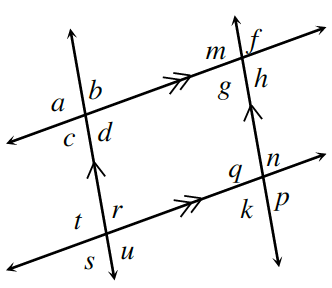### Home > CCG > Chapter 6 > Lesson 6.2.5 > Problem6-94

6-94.Examine the angles formed by parallel lines at right.

1. If $r=5x+3º$ and $k=4x+9º$, solve for $x$. Justify your answer.

How are $r$ and $k$ related? How can you use this relationship to solve for $x$?

$x=6º$

2. If $c=114º$, what is $q$? Justify your answer.

Does $c$ relate to another angle that might help you determine the measure of $q$?

Relate $c$, $t$, $r$, or $g$.

3. If $g=88º$, then what is $q$? Justify your answer.

What is the relationship between $g$ and $q$? How does this help you determine the measure of $q$?